## The Binary Octahedral GroupThe complex numbers together with infinity form a sphere called
the Riemann sphere. The 6 simplest numbers on this sphere lie at points we could call the north pole, the south pole, the east pole, the west pole, the front pole and the back pole. They’re the corners of an octahedron!On the Earth, I’d say the “front pole” is where the prime meridian meets the equator at 0°N 0°E. It’s called Null Island, but there’s no island there—just a buoy. Here it is:Where’s the back pole, the east pole and the west pole? I’ll leave two of these as puzzles, but I discovered that in Singapore I’m fairly close to the east pole: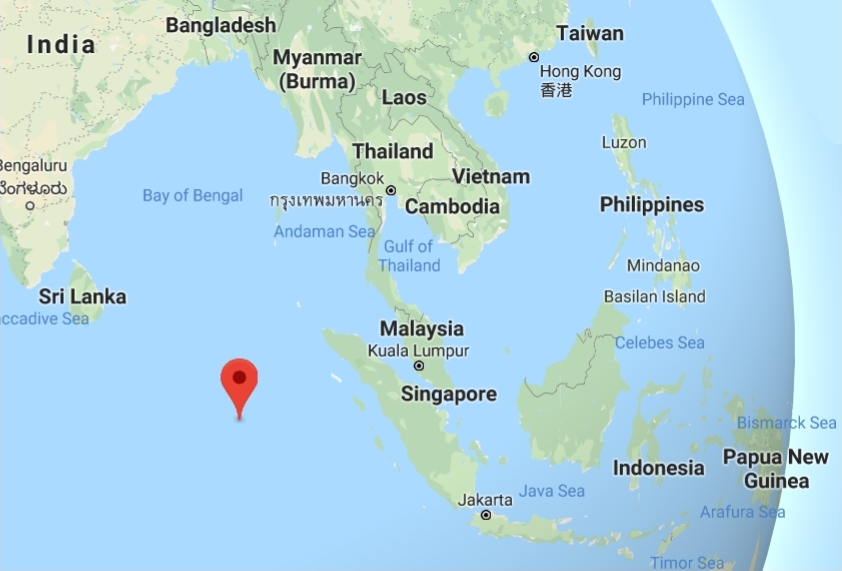If you think of the octahedron’s corners as the quaternions$\pm i, \pm j, \pm k,$ you can look for unit quaternions$q$ such that whenever$x$ is one of these corners, so is$qxq^{-1}$. There are 48 of these! They form a group called the binary octahedral group.

By how we set it up, the binary octahedral group acts as rotational symmetries of the octahedron: any transformation sending$x$ to$qxq^{-1}$ is a rotation. But this group is a double cover of the octahedron’s rotational symmetry group! That is, pairs of elements of the binary octahedral group describe the same rotation of the octahedron.

If we go back and think of the Earth’s 6 poles as points$0, \pm 1,\pm i, \infty$ on the Riemann sphere instead of$\pm i, \pm j, \pm k$, we can think of the binary octahedral group as a subgroup of$\mathrm{SL}(2,\mathbb{C}$), since this acts as conformal transformations of the Riemann sphere!

If we do this, the binary octahedral group is actually a subgroup of$\mathrm{SU}(2)$, the double cover of the rotation group—which is isomorphic to the group of unit quaternions. So it all hangs together.

It’s fun to actualy see the unit quaternions in the binary octahedral group. First we have 8 that form the corners of a cross-polytope (the 4d analogue of an octahedron):$\pm 1, \pm i , \pm j , \pm k$

These form a group on their own, called the quaternion group. Then we have 16 that form the corners of a hypercube (the 4d analogue of a cube, also called a tesseract or 4-cube):$\displaystyle{ \frac{\pm 1 \pm i \pm j \pm k}{2} }$

These don’t form a group, but if we take them together with the 8 previous ones we get a 24-element subgroup of the unit quaternions called the binary tetrahedral group. They’re also the vertices of a 24-cell, which is yet another highly symmetrical shape in 4 dimensions (a 4-dimensional regular polytope that doesn’t have a 3d analogue).

That accounts for half the quaternions in the binary octahedral group! Here are the other 24:$\displaystyle{ \frac{\pm 1 \pm i}{\sqrt{2}}, \frac{\pm 1 \pm j}{\sqrt{2}}, \frac{\pm 1 \pm k}{\sqrt{2}}, }$$\displaystyle{ \frac{\pm i \pm j}{\sqrt{2}}, \frac{\pm j \pm k}{\sqrt{2}}, \frac{\pm k \pm i}{\sqrt{2}} }$

These form the vertices of another 24-cell!

The first 24 quaternions, those in the binary tetrahedral group, give rotations that preserve each one of the two tetrahedra that you can fit around an octahedron like this: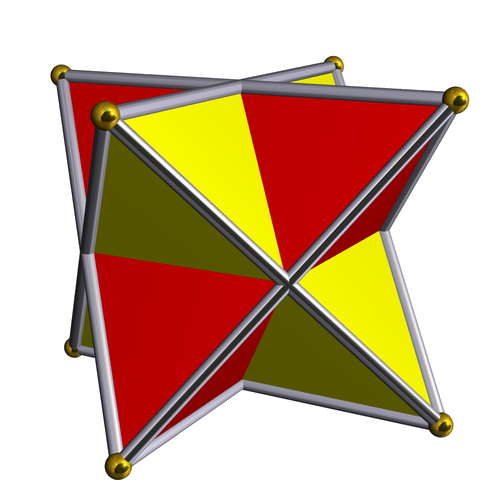while the second 24 switch these tetrahedra.

The 6 elements$\pm i , \pm j , \pm k$

describe 180° rotations around the octahedron’s 3 axes, the 16 elements$\displaystyle{ \frac{\pm 1 \pm i \pm j \pm k}{2} }$

describe 120° clockwise rotations of the octahedron’s 8 triangles, the 12 elements$\displaystyle{ \frac{\pm 1 \pm i}{\sqrt{2}}, \frac{\pm 1 \pm j}{\sqrt{2}}, \frac{\pm 1 \pm k}{\sqrt{2}} }$

describe 90° clockwise rotations holding fixed one of the octahedron’s 6 vertices, and the 12 elements$\displaystyle{ \frac{\pm i \pm j}{\sqrt{2}}, \frac{\pm j \pm k}{\sqrt{2}}, \frac{\pm k \pm i}{\sqrt{2}} }$

describe 180° clockwise rotations of the octahedron’s 6 opposite pairs of edges.

Finally, the two elements$\pm 1$

do nothing!

So, we can have a lot of fun with the idea that a sphere has 6 poles.

### 12 Responses to The Binary Octahedral Group

1.Greg Egan says:

Here’s a picture of the 24-cell:One further nice twist in all this symmetry is that pairs of vertices, and pairs of cell-centres, of the 24-cell act as 4D rotations that are symmetries of the 24-cell itself!

•John Baez says:

Nice! Is the color the 4th coordinate?

When talking to Tim Silverman on the n-Cafeé, I learned that in addition to being able to partition the vertices of the 24-cell into those of a hypercube and those of a cross-polytope (24 = 16 + 8), as explained above, we could also partition them into the vertices of 3 cross-polytopes (24 = 8 + 8 + 8), because in 4 dimensions we can partition the vertices of the hypercube into the vertices of 2 cross-polytopes (16 = 8 + 8). This is a nice “echo” of how we can partition the vertices of the cube into the vertices of 2 tetrahedra (8 = 4 + 4), a fact which lets us fit the binary tetrahedral group into the binary octahedral group, giving a way to put two copies the vertices of the 24-cell into the 48-vertex shape I’m discussing above (48 = 24 + 24). It’s all very tightly wound!

If you wanted to have fun coloring the vertices of your animation in a different way you could try breaking the 24 vertices into 3 different cross-polytopes (24 = 8 + 8 + 8), or a hypercube and a cross-polytope (24 = 16 + 8).

I did the latter in my own low-tech way here. Cross-polytope:Hypercube: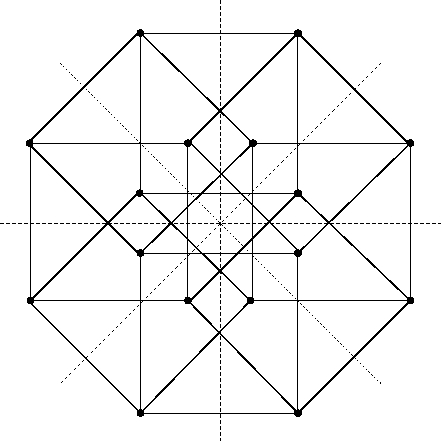24-cell, formed by overlaying the cross-polytope and hypercube:Another fun thing I noticed while writing this post is that you get the vertices of a 24-cell by taking all permutations of (±1, ±1, 0, 0). These days I deal with bouts of insomnia by trying to visualize this. It’s like a 4-dimensional version of how the cuboctahedron has vertices that are permutations of (±1, ±1, 0). To get this cuboctahedron, you can take a cube with vertices (±1, ±1, ±1) and cut off its corners in a suitable way, replacing them by equilateral triangles whose corners lie on the 3 coordinate planes. To get the 24-cell, you can take the hypercube with vertices (±1, ±1, ±1, ±1) and hack off its corners in a more complicated way, replacing one with an octahedron! (If you just truncate the hypercube you replace each corner with a tetrahedron—that’s not what we need here.)

I’m not sure if visualizing these things helps me fall asleep or keeps me awake, but it’s a lot more fun than thinking about all the work I should be doing and how the world is going to hell.)

2.Greg Egan says:

Here’s a version that partitions the vertices into three cross-polytopes, and also marks out one of the octahedral cells in black to make it easier to follow.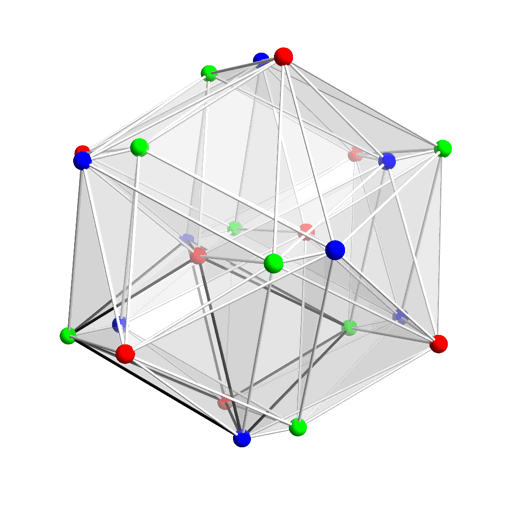The colours in the previous image were a bit of a trick; I wanted to make two octahedral cells visible by distinguishing them, but I also wanted to exploit the symmetry of the figure in order to show only half a rotation and make the GIF smaller. So the hues aren’t strictly linked to any coordinate, but just depend on how much the figure has been rotated, in such a way that the two cells swap colours after half a rotation.

3.arch1 says:

Thank you John and Greg.
Greg, do your pictures show a 2-D parallel projection of a 24-cell rotating in 4-space around a fixed axis (so basically you’re just dropping two coordinates before displaying)? If so, is there any special relationship between the (planar) rotation axis and the plane of projection, other than the fact that they’re not equal?

•Greg Egan says:

The rotations of these figures in 4D are of a type that doesn’t leave any point on the 3-sphere fixed, but moves them all with equal velocity; you get that kind of rotation by combining rotations by equal angles in two orthogonal planes.

The projection is just something generic that I chose by trial and error to look OK throughout the course of the rotation.

4.arch1 says:

Thanks Greg.

5.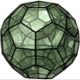jasonhise64 says:

If you explore the surface of a hypercube you can identify vertices of the first 24-cell as room centers + corners, and those of the second 24-cell with the midpoints of each wall between rooms!

•John Baez says:

Yes, that’s very nice! The room centers form the vertices of the cross-polytope dual to the hypercube; together they form the vertices of a 24-cell. The midpoints of the walls of the cube form the vertices of the dual 24-cell. Interestingly there doesn’t seem to be a canonical way to partition these vertices into those of a hypercube and a 24-cells, given the structure we have so far.

6.Hendrik Boom says:

Didn’t Winnie the Pooh discover the east pole?

•John Baez says:

That sounds vaguely familiar, but it’s been a long time since I’ve read that book!

7.Blake Stacey says:

Pointing back to a discussion from February: the binary tetrahedral group is the fiducial stabilizer of the Hesse SIC.

The eigenstates of the three Pauli operators form a regular octahedron inscribed in the Bloch sphere. Transformations of the qubit state space that permute the vertices of this octahedron should correspond to elements of the binary octahedral group. Offhand, though, I can’t recall of facts about the binary octahedral group being used to prove things in quantum information. I think that when people mod out the phase freedom in the qubit Clifford group to get a discrete group, they go all the way and end up with the symmetries of a regular octahedron, which are just the symmetries of a cube.

8. The E8 lattice is a thing of beauty, taking full advantage of the magic properties of the number 8. The octahedron has 8 sides. Wouldn’t it be cool if you could build the E8 lattice from the humble octahedron?

David Harden thinks he’s found a way! But I haven’t carefully checked that it works: it takes some calculations. Can you check it?

This site uses Akismet to reduce spam. Learn how your comment data is processed.